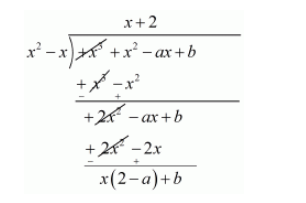# If f(x) = x3 + x2 − ax + b is divisible`
Question:

If $l(x)=x^{3}+x^{2}-a x+b$ is divisible by $x^{2}-x$ write the value of $a$ and $b$.

Solution:

We are given $f(x)=x^{3}+x^{2}-a x+b$ is exactly divisible by $x^{2}-x$ then the remainder should be zeroTherefore Quotient $=x+2$ and

Remainder $=x(2-a)+b$

Now, Remainder $=0$

$x(2-a)+b=0$

$x(2-a)+b=0 x+0$

Equating coefficient of $\mathrm{x}$, we get

$2-a=0$

$2=a$

Equating constant term

$b=0$

Hence, the value of $a$ and $b$ are $a=2, b=0$